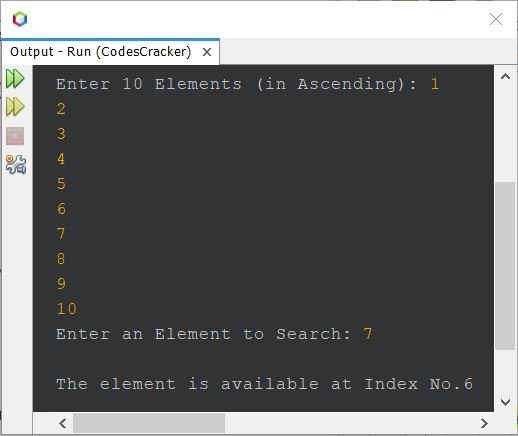# Java Program - Binary Search

If you're not aware about, How binary search works ?
Then refer to Binary Search Logic and Algorithm. Now let's move on, and create a program in Java for binary search.

## Binary Search in Java - Basic Version

The question is, write a Java program to perform binary search based on 10 elements. All the 10 elements and the element to search using binary search, must be received by user at run-time of the program. The program given below is the answer to this question:

```import java.util.Scanner;

public class CodesCracker
{
public static void main(String[] args)
{
int size=10, i, search, first, last, middle;
int[] arr = new int[size];
Scanner scan = new Scanner(System.in);

System.out.print("Enter 10 Elements (in Ascending): ");
for(i=0; i<size; i++)
{
arr[i] = scan.nextInt();
}
System.out.print("Enter an Element to Search: ");
search = scan.nextInt();

first = 0;
last = size-1;
middle = (first+last)/2;

while(first<=last)
{
if(arr[middle]<search)
{
first = middle+1;
}
else if(arr[middle]==search)
{
System.out.println("\nThe element is available at Index No." +middle);
break;
}
else
{
last = middle-1;
}
middle = (first+last)/2;
}

if(first>last)
{
System.out.println("\nThe element is not available in given array");
}
}
}```

The snapshot given below shows the sample run of above Java program on binary search, with user input 1, 2, 3, 4, 5, 6, 7, 8, 9, 10 as ten elements and 7 as element to search:## Binary Search in Java - Complete Version

Since previous program has some limitations like when user enters elements in random order, then the program produces incorrect output. Also, the program allows user to enter only 10 elements. Therefore I've modified that program, to remove these limitations. Here is the modified version of previous program.

```import java.util.Scanner;

public class CodesCracker
{
public static void main(String[] args)
{
int tot, i, search, first, last, middle, j, x;
Scanner scan = new Scanner(System.in);

System.out.print("Enter the Size: ");
tot = scan.nextInt();

int[] arr = new int[tot];

System.out.print("Enter " +tot+" Elements: ");
for(i=0; i<tot; i++)
arr[i] = scan.nextInt();

// following block of code, sorts the array
for(i=0; i<(tot-1); i++)
{
for(j=0; j<(tot-i-1); j++)
{
if(arr[j]>arr[j+1])
{
x = arr[j];
arr[j] = arr[j+1];
arr[j+1] = x;
}
}
}

// following block of code, prints the sorted array
System.out.println("\nNow the sorted array is:");
for(i=0; i<tot; i++)
System.out.print(arr[i]+ " ");

// things are done, now let's do binary search
System.out.print("\n\nEnter an Element to Search: ");
search = scan.nextInt();

first = 0;
last = tot-1;
middle = (first+last)/2;

while(first<=last)
{
if(arr[middle]<search)
first = middle+1;
else if(arr[middle]==search)
{
System.out.println("\nThe element found at Index No." +middle+ ", Position No." +(middle+1));
break;
}
else
last = middle-1;

middle = (first+last)/2;
}

if(first>last)
System.out.println("\nThe element is not available in given array");
}
}```

The sample run of above program with user inputs 6 as size, 100, 101, 102, 103, 104, 105 as its six elements, and 103 as element to search:## Binary Search in Java using for Loop

To create a program in Java that performs binary search, completely using for loop, then replace the following block of code, from above program:

```first = 0;
last = size-1;
middle = (first+last)/2;

while(first<=last)
{
if(arr[middle]<elem)
first = middle+1;
else if(arr[middle]==elem)
{
System.out.println("\nThe element found at Index No." +middle+ ", Position No." +(middle+1));
break;
}
else
last = middle-1;

middle = (first+last)/2;
}```

with the block of code, given below:

```first = 0;
last = size-1;

for(middle=(first+last)/2; first<=last; middle=(first+last)/2)
{
if(arr[middle]<elem)
first = middle+1;
else if(arr[middle]==elem)
{
System.out.println("\nThe element found at Index No." +middle+ ", Position No." +(middle+1));
break;
}
else
last = middle-1;
}```

Also, you can put the first two statements, inside the first statement of for loop, separate by comma. That is,

`for(first=0, last=(size-1), middle=(first+last)/2; first<=last; middle=(first+last)/2)`

#### Same Program in Other Languages

Java Online Test

« Previous Program Next Program »